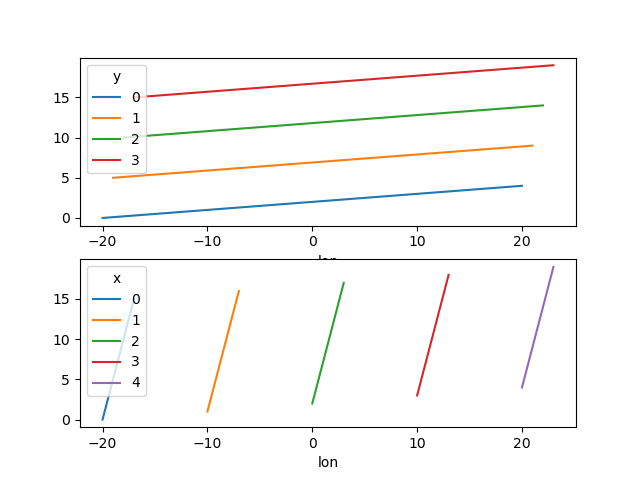# Plotting¶

## Introduction¶

Labeled data enables expressive computations. These same labels can also be used to easily create informative plots.

xarray’s plotting capabilities are centered around DataArray objects. To plot Dataset objects simply access the relevant DataArrays, i.e. dset['var1']. Dataset specific plotting routines are also available (see Datasets). Here we focus mostly on arrays 2d or larger. If your data fits nicely into a pandas DataFrame then you’re better off using one of the more developed tools there.

xarray plotting functionality is a thin wrapper around the popular matplotlib library. Matplotlib syntax and function names were copied as much as possible, which makes for an easy transition between the two. Matplotlib must be installed before xarray can plot.

To use xarray’s plotting capabilities with time coordinates containing cftime.datetime objects nc-time-axis v1.2.0 or later needs to be installed.

For more extensive plotting applications consider the following projects:

• Seaborn: “provides a high-level interface for drawing attractive statistical graphics.” Integrates well with pandas.

• HoloViews and GeoViews: “Composable, declarative data structures for building even complex visualizations easily.” Includes native support for xarray objects.

• hvplot: hvplot makes it very easy to produce dynamic plots (backed by Holoviews or Geoviews) by adding a hvplot accessor to DataArrays.

• Cartopy: Provides cartographic tools.

### Imports¶

The following imports are necessary for all of the examples.

In : import numpy as np

In : import pandas as pd

In : import matplotlib.pyplot as plt

In : import xarray as xr


For these examples we’ll use the North American air temperature dataset.

In : airtemps = xr.tutorial.open_dataset("air_temperature")

In : airtemps
Out:
<xarray.Dataset>
Dimensions:  (lat: 25, lon: 53, time: 2920)
Coordinates:
* lat      (lat) float32 75.0 72.5 70.0 67.5 65.0 ... 25.0 22.5 20.0 17.5 15.0
* lon      (lon) float32 200.0 202.5 205.0 207.5 ... 322.5 325.0 327.5 330.0
* time     (time) datetime64[ns] 2013-01-01 ... 2014-12-31T18:00:00
Data variables:
air      (time, lat, lon) float32 ...
Attributes:
Conventions:  COARDS
title:        4x daily NMC reanalysis (1948)
description:  Data is from NMC initialized reanalysis\n(4x/day).  These a...
platform:     Model
references:   http://www.esrl.noaa.gov/psd/data/gridded/data.ncep.reanaly...

# Convert to celsius
In : air = airtemps.air - 273.15

# copy attributes to get nice figure labels and change Kelvin to Celsius
In : air.attrs = airtemps.air.attrs

In : air.attrs["units"] = "deg C"


Note

Until GH1614 is solved, you might need to copy over the metadata in attrs to get informative figure labels (as was done above).

## DataArrays¶

### One Dimension¶

#### Simple Example¶

The simplest way to make a plot is to call the DataArray.plot() method.

In : air1d = air.isel(lat=10, lon=10)

In : air1d.plot()
Out: [<matplotlib.lines.Line2D at 0x7f832eccefa0>]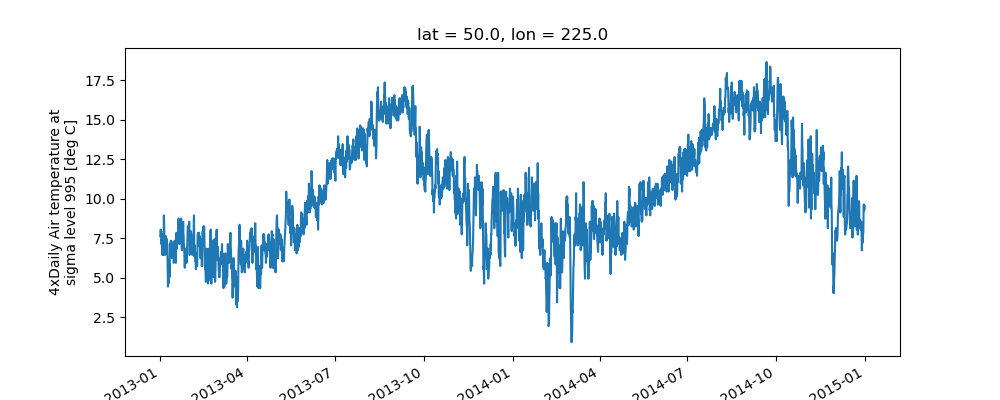xarray uses the coordinate name along with metadata attrs.long_name, attrs.standard_name, DataArray.name and attrs.units (if available) to label the axes. The names long_name, standard_name and units are copied from the CF-conventions spec. When choosing names, the order of precedence is long_name, standard_name and finally DataArray.name. The y-axis label in the above plot was constructed from the long_name and units attributes of air1d.

In : air1d.attrs
Out:
{'long_name': '4xDaily Air temperature at sigma level 995',
'units': 'deg C',
'precision': 2,
'GRIB_id': 11,
'GRIB_name': 'TMP',
'var_desc': 'Air temperature',
'dataset': 'NMC Reanalysis',
'level_desc': 'Surface',
'statistic': 'Individual Obs',
'parent_stat': 'Other',
'actual_range': array([185.16, 322.1 ], dtype=float32)}


Additional arguments are passed directly to the matplotlib function which does the work. For example, xarray.plot.line() calls matplotlib.pyplot.plot passing in the index and the array values as x and y, respectively. So to make a line plot with blue triangles a matplotlib format string can be used:

In : air1d[:200].plot.line("b-^")
Out: [<matplotlib.lines.Line2D at 0x7f832ec89e50>]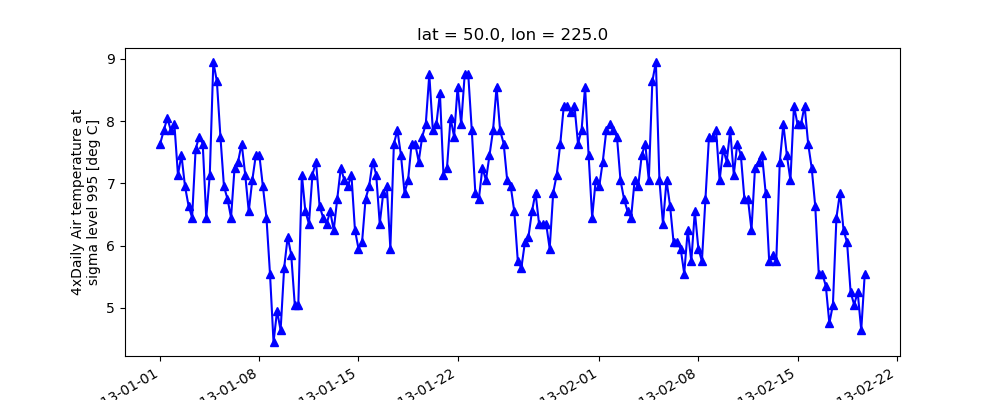Note

Not all xarray plotting methods support passing positional arguments to the wrapped matplotlib functions, but they do all support keyword arguments.

Keyword arguments work the same way, and are more explicit.

In : air1d[:200].plot.line(color="purple", marker="o")
Out: [<matplotlib.lines.Line2D at 0x7f83347f5b80>]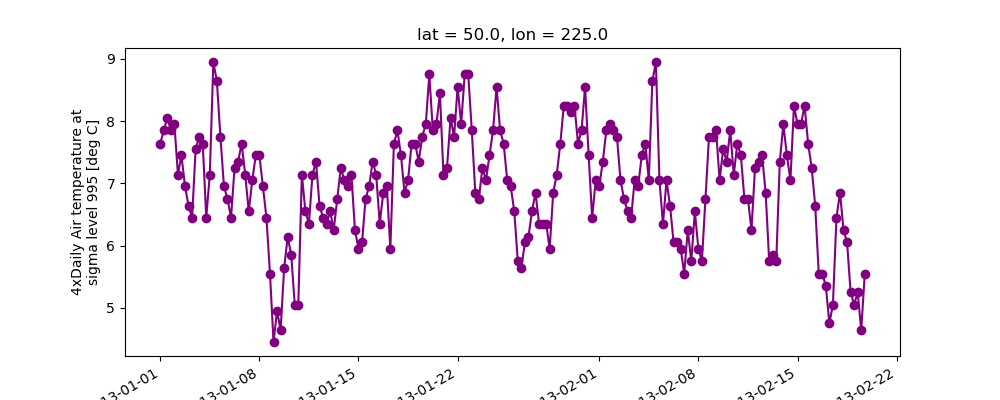To add the plot to an existing axis pass in the axis as a keyword argument ax. This works for all xarray plotting methods. In this example axes is an array consisting of the left and right axes created by plt.subplots.

In : fig, axes = plt.subplots(ncols=2)

In : axes
Out: array([<AxesSubplot:>, <AxesSubplot:>], dtype=object)

In : air1d.plot(ax=axes)
Out: [<matplotlib.lines.Line2D at 0x7f832ebed580>]

In : air1d.plot.hist(ax=axes)
Out:
(array([  9.,  38., 255., 584., 542., 489., 368., 258., 327.,  50.]),
array([ 0.9500122,  2.7190125,  4.488013 ,  6.257013 ,  8.026013 ,
9.795013 , 11.5640135, 13.333014 , 15.102015 , 16.871014 ,
18.640015 ], dtype=float32),
<BarContainer object of 10 artists>)

In : plt.tight_layout()

In : plt.draw()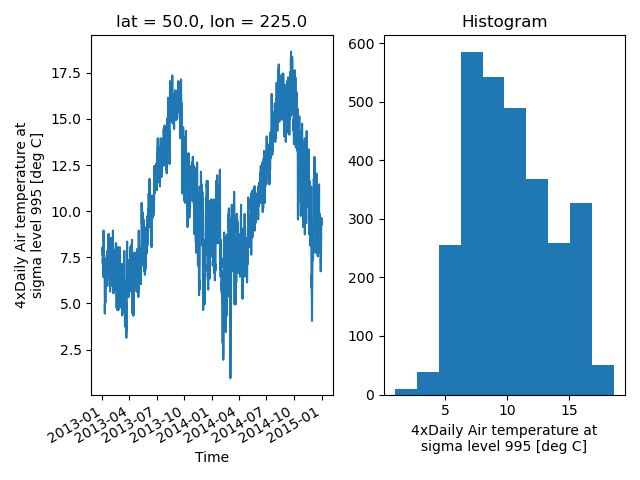On the right is a histogram created by xarray.plot.hist().

#### Controlling the figure size¶

You can pass a figsize argument to all xarray’s plotting methods to control the figure size. For convenience, xarray’s plotting methods also support the aspect and size arguments which control the size of the resulting image via the formula figsize = (aspect * size, size):

In : air1d.plot(aspect=2, size=3)
Out: [<matplotlib.lines.Line2D at 0x7f8334872c10>]

In : plt.tight_layout()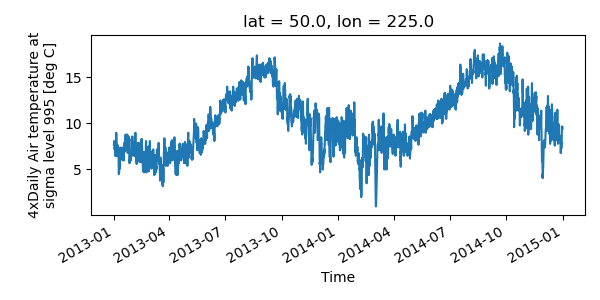This feature also works with Faceting. For facet plots, size and aspect refer to a single panel (so that aspect * size gives the width of each facet in inches), while figsize refers to the entire figure (as for matplotlib’s figsize argument).

Note

If figsize or size are used, a new figure is created, so this is mutually exclusive with the ax argument.

Note

The convention used by xarray (figsize = (aspect * size, size)) is borrowed from seaborn: it is therefore not equivalent to matplotlib’s.

#### Determine x-axis values¶

Per default dimension coordinates are used for the x-axis (here the time coordinates). However, you can also use non-dimension coordinates, MultiIndex levels, and dimensions without coordinates along the x-axis. To illustrate this, let’s calculate a ‘decimal day’ (epoch) from the time and assign it as a non-dimension coordinate:

In : decimal_day = (air1d.time - air1d.time) / pd.Timedelta("1d")

In : air1d_multi = air1d.assign_coords(decimal_day=("time", decimal_day))

In : air1d_multi
Out:
<xarray.DataArray 'air' (time: 2920)>
array([7.6400146, 7.850006 , 8.049988 , ..., 9.339996 , 9.339996 ,
9.540009 ], dtype=float32)
Coordinates:
lat          float32 50.0
lon          float32 225.0
* time         (time) datetime64[ns] 2013-01-01 ... 2014-12-31T18:00:00
decimal_day  (time) float64 0.0 0.25 0.5 0.75 ... 729.0 729.2 729.5 729.8
Attributes:
long_name:     4xDaily Air temperature at sigma level 995
units:         deg C
precision:     2
GRIB_id:       11
GRIB_name:     TMP
var_desc:      Air temperature
dataset:       NMC Reanalysis
level_desc:    Surface
statistic:     Individual Obs
parent_stat:   Other
actual_range:  [185.16 322.1 ]


To use 'decimal_day' as x coordinate it must be explicitly specified:

In : air1d_multi.plot(x="decimal_day")
Out: [<matplotlib.lines.Line2D at 0x7f833474da90>]


Creating a new MultiIndex named 'date' from 'time' and 'decimal_day', it is also possible to use a MultiIndex level as x-axis:

In : air1d_multi = air1d_multi.set_index(date=("time", "decimal_day"))

In : air1d_multi.plot(x="decimal_day")
Out: [<matplotlib.lines.Line2D at 0x7f83347bfac0>]


Finally, if a dataset does not have any coordinates it enumerates all data points:

In : air1d_multi = air1d_multi.drop("date")

In : air1d_multi.plot()
Out: [<matplotlib.lines.Line2D at 0x7f83347bf3d0>]


The same applies to 2D plots below.

#### Multiple lines showing variation along a dimension¶

It is possible to make line plots of two-dimensional data by calling xarray.plot.line() with appropriate arguments. Consider the 3D variable air defined above. We can use line plots to check the variation of air temperature at three different latitudes along a longitude line:

In : air.isel(lon=10, lat=[19, 21, 22]).plot.line(x="time")
Out:
[<matplotlib.lines.Line2D at 0x7f83347a04c0>,
<matplotlib.lines.Line2D at 0x7f83348b7e80>,
<matplotlib.lines.Line2D at 0x7f83349101c0>]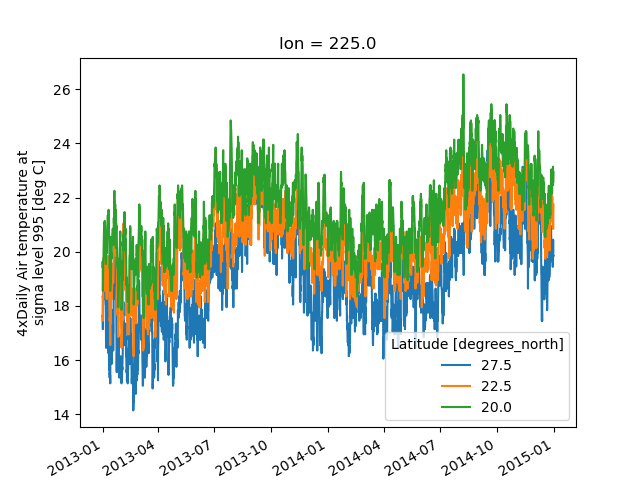It is required to explicitly specify either

1. x: the dimension to be used for the x-axis, or

2. hue: the dimension you want to represent by multiple lines.

Thus, we could have made the previous plot by specifying hue='lat' instead of x='time'. If required, the automatic legend can be turned off using add_legend=False. Alternatively, hue can be passed directly to xarray.plot.line() as air.isel(lon=10, lat=[19,21,22]).plot.line(hue=’lat’).

#### Dimension along y-axis¶

It is also possible to make line plots such that the data are on the x-axis and a dimension is on the y-axis. This can be done by specifying the appropriate y keyword argument.

In : air.isel(time=10, lon=[10, 11]).plot(y="lat", hue="lon")
Out:
[<matplotlib.lines.Line2D at 0x7f8326b5a4f0>,
<matplotlib.lines.Line2D at 0x7f8326b5a5b0>]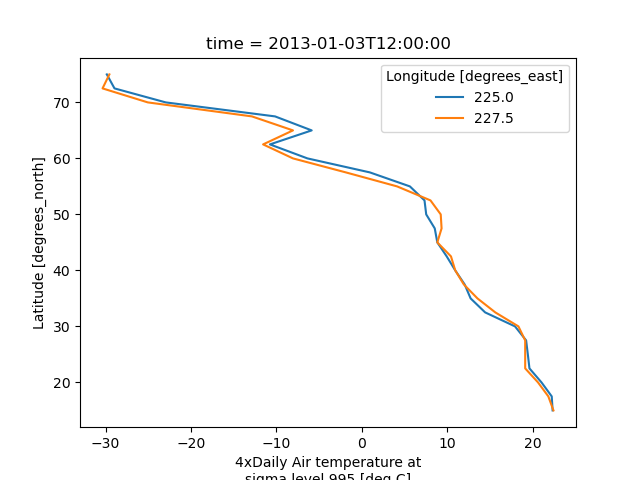#### Step plots¶

As an alternative, also a step plot similar to matplotlib’s plt.step can be made using 1D data.

In : air1d[:20].plot.step(where="mid")
Out: [<matplotlib.lines.Line2D at 0x7f8326af89a0>]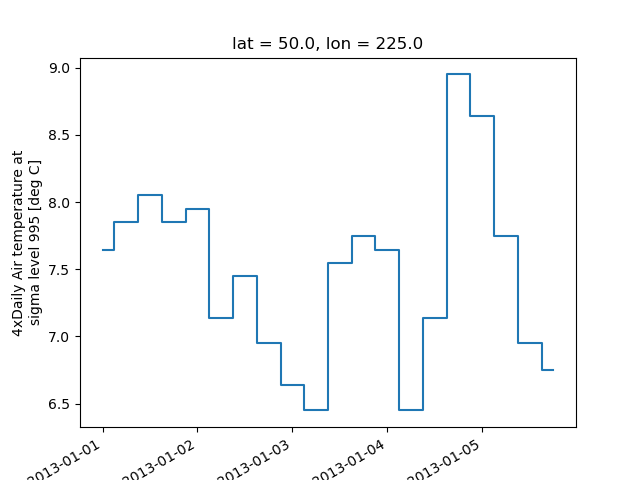The argument where defines where the steps should be placed, options are 'pre' (default), 'post', and 'mid'. This is particularly handy when plotting data grouped with Dataset.groupby_bins().

In : air_grp = air.mean(["time", "lon"]).groupby_bins("lat", [0, 23.5, 66.5, 90])

In : air_mean = air_grp.mean()

In : air_std = air_grp.std()

In : air_mean.plot.step()

In : (air_mean + air_std).plot.step(ls=":")

In : (air_mean - air_std).plot.step(ls=":")

In : plt.ylim(-20, 30)
Out: (-20.0, 30.0)

In : plt.title("Zonal mean temperature")
Out: Text(0.5, 1.0, 'Zonal mean temperature')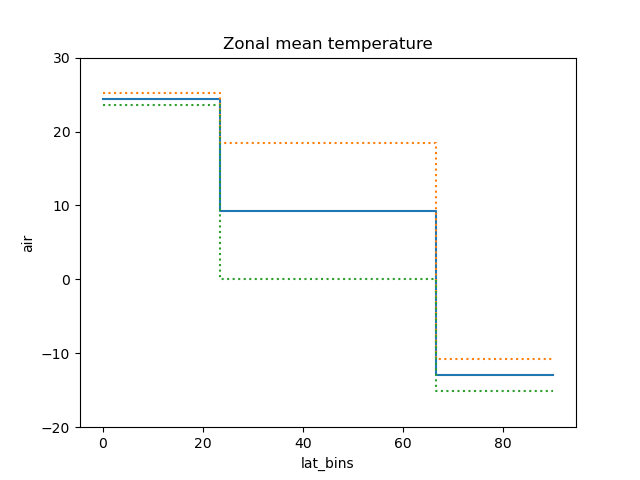In this case, the actual boundaries of the bins are used and the where argument is ignored.

### Other axes kwargs¶

The keyword arguments xincrease and yincrease let you control the axes direction.

In : air.isel(time=10, lon=[10, 11]).plot.line(
....:     y="lat", hue="lon", xincrease=False, yincrease=False
....: )
....:
Out:
[<matplotlib.lines.Line2D at 0x7f8326a6a910>,
<matplotlib.lines.Line2D at 0x7f8326a6a970>]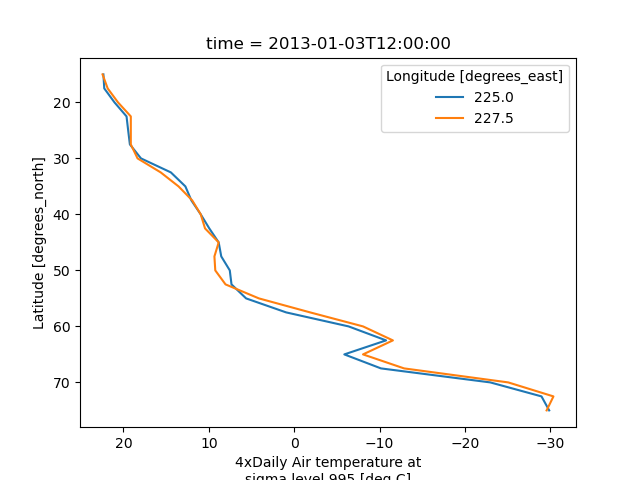In addition, one can use xscale, yscale to set axes scaling; xticks, yticks to set axes ticks and xlim, ylim to set axes limits. These accept the same values as the matplotlib methods Axes.set_(x,y)scale(), Axes.set_(x,y)ticks(), Axes.set_(x,y)lim() respectively.

### Two Dimensions¶

#### Simple Example¶

The default method DataArray.plot() calls xarray.plot.pcolormesh() by default when the data is two-dimensional.

In : air2d = air.isel(time=500)

In : air2d.plot()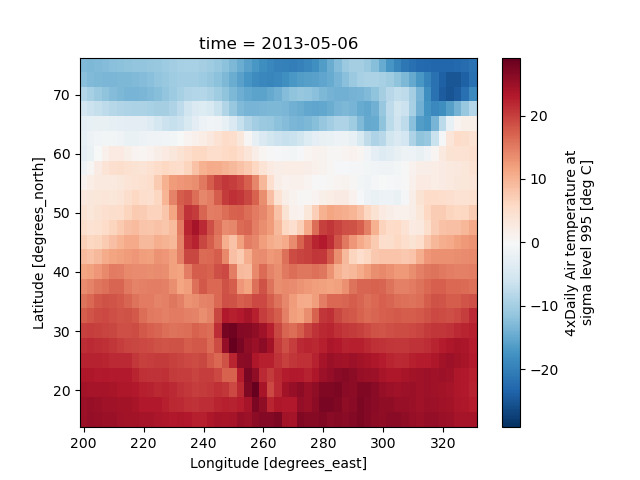All 2d plots in xarray allow the use of the keyword arguments yincrease and xincrease.

In : air2d.plot(yincrease=False)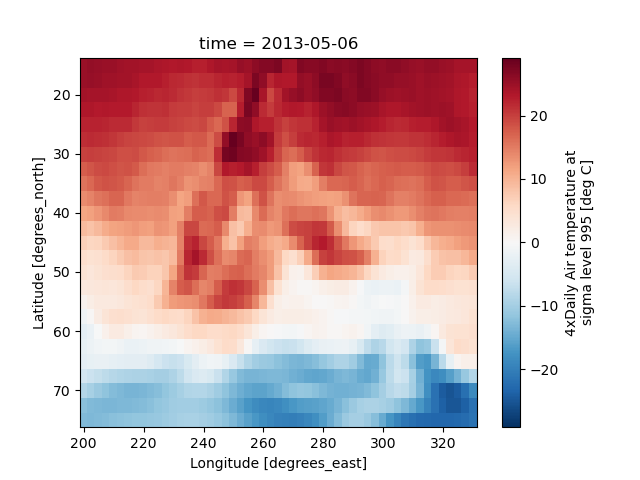Note

We use xarray.plot.pcolormesh() as the default two-dimensional plot method because it is more flexible than xarray.plot.imshow(). However, for large arrays, imshow can be much faster than pcolormesh. If speed is important to you and you are plotting a regular mesh, consider using imshow.

#### Missing Values¶

xarray plots data with Missing values.

In : bad_air2d = air2d.copy()

In : bad_air2d[dict(lat=slice(0, 10), lon=slice(0, 25))] = np.nan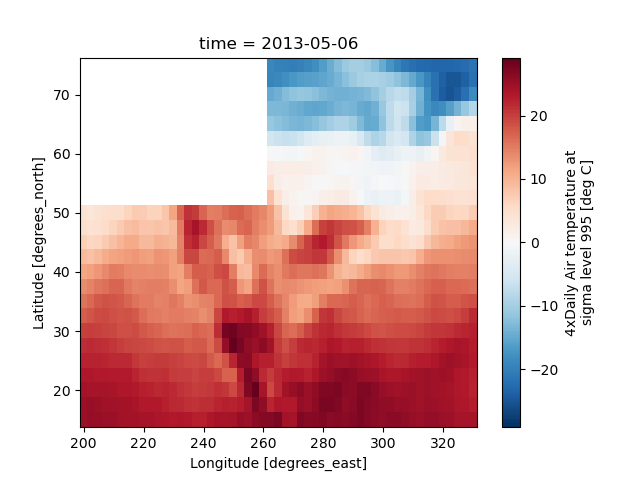#### Nonuniform Coordinates¶

It’s not necessary for the coordinates to be evenly spaced. Both xarray.plot.pcolormesh() (default) and xarray.plot.contourf() can produce plots with nonuniform coordinates.

In : b = air2d.copy()

# Apply a nonlinear transformation to one of the coords
In : b.coords["lat"] = np.log(b.coords["lat"])

In : b.plot()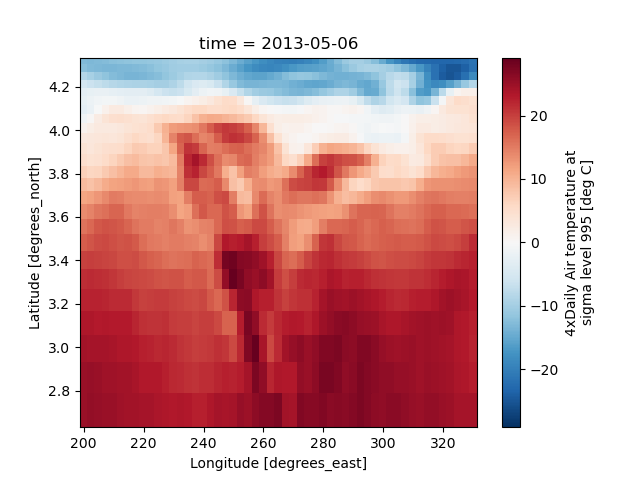#### Calling Matplotlib¶

Since this is a thin wrapper around matplotlib, all the functionality of matplotlib is available.

In : air2d.plot(cmap=plt.cm.Blues)

In : plt.title("These colors prove North America\nhas fallen in the ocean")
Out: Text(0.5, 1.0, 'These colors prove North America\nhas fallen in the ocean')

In : plt.ylabel("latitude")
Out: Text(0, 0.5, 'latitude')

In : plt.xlabel("longitude")
Out: Text(0.5, 0, 'longitude')

In : plt.tight_layout()

In : plt.draw()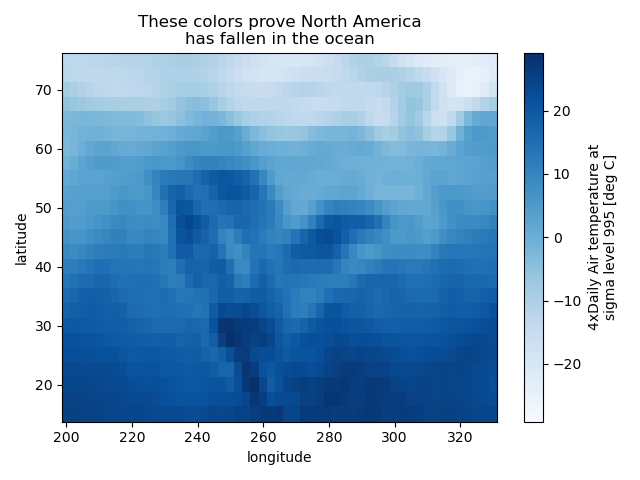Note

xarray methods update label information and generally play around with the axes. So any kind of updates to the plot should be done after the call to the xarray’s plot. In the example below, plt.xlabel effectively does nothing, since d_ylog.plot() updates the xlabel.

In : plt.xlabel("Never gonna see this.")
Out: Text(0.5, 0, 'Never gonna see this.')

In : air2d.plot()

In : plt.draw()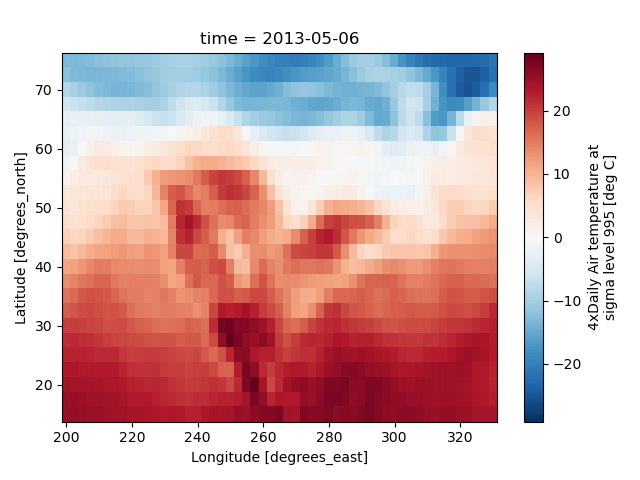#### Colormaps¶

xarray borrows logic from Seaborn to infer what kind of color map to use. For example, consider the original data in Kelvins rather than Celsius:

In : airtemps.air.isel(time=0).plot()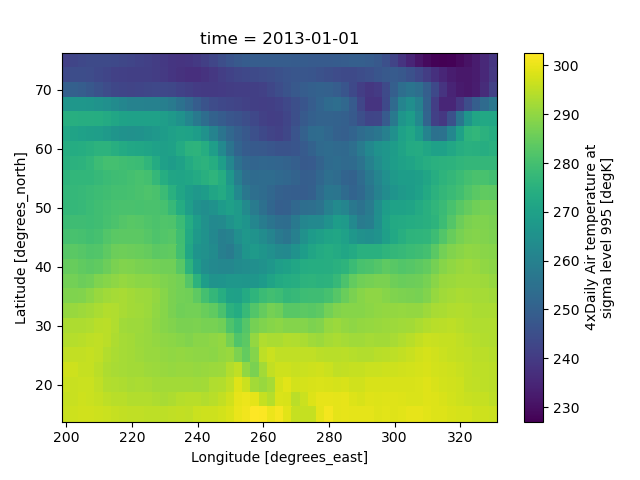The Celsius data contain 0, so a diverging color map was used. The Kelvins do not have 0, so the default color map was used.

#### Robust¶

Outliers often have an extreme effect on the output of the plot. Here we add two bad data points. This affects the color scale, washing out the plot.

In : air_outliers = airtemps.air.isel(time=0).copy()

In : air_outliers[0, 0] = 100

In : air_outliers[-1, -1] = 400

In : air_outliers.plot()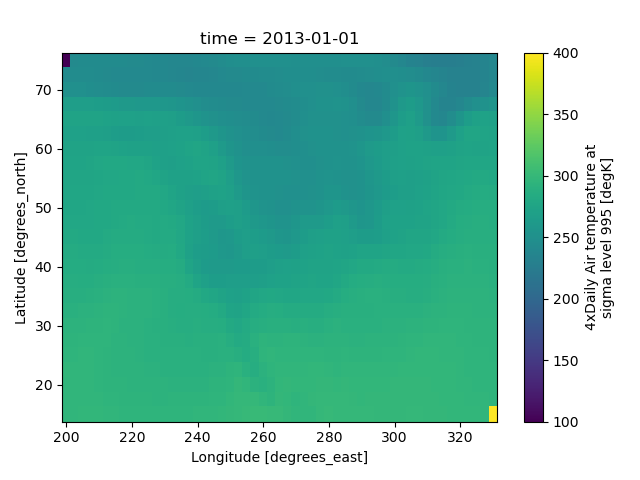This plot shows that we have outliers. The easy way to visualize the data without the outliers is to pass the parameter robust=True. This will use the 2nd and 98th percentiles of the data to compute the color limits.

In : air_outliers.plot(robust=True)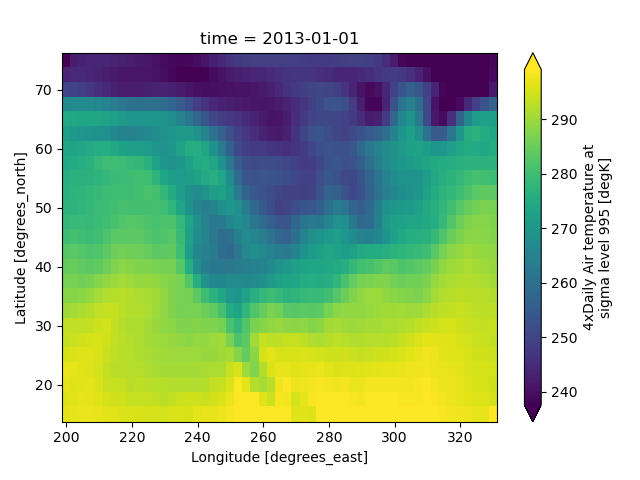Observe that the ranges of the color bar have changed. The arrows on the color bar indicate that the colors include data points outside the bounds.

#### Discrete Colormaps¶

It is often useful, when visualizing 2d data, to use a discrete colormap, rather than the default continuous colormaps that matplotlib uses. The levels keyword argument can be used to generate plots with discrete colormaps. For example, to make a plot with 8 discrete color intervals:

In : air2d.plot(levels=8)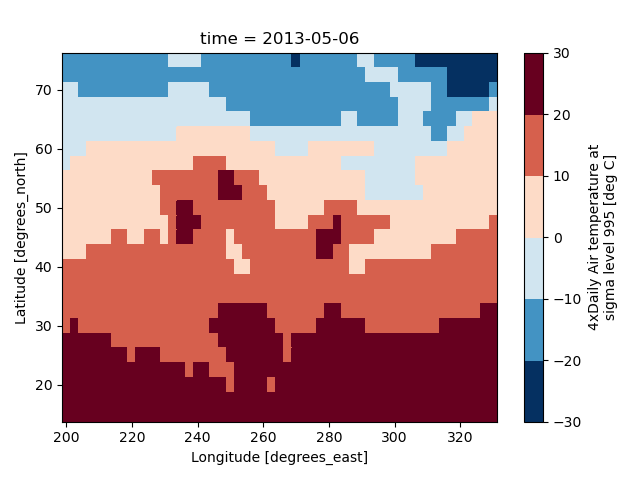It is also possible to use a list of levels to specify the boundaries of the discrete colormap:

In : air2d.plot(levels=[0, 12, 18, 30])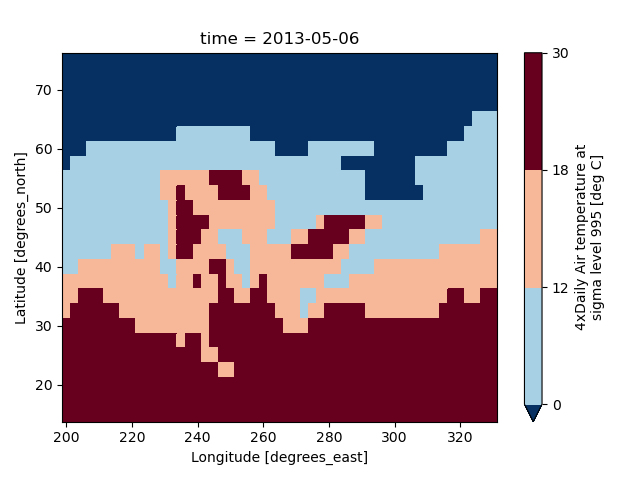You can also specify a list of discrete colors through the colors argument:

In : flatui = ["#9b59b6", "#3498db", "#95a5a6", "#e74c3c", "#34495e", "#2ecc71"]

In : air2d.plot(levels=[0, 12, 18, 30], colors=flatui)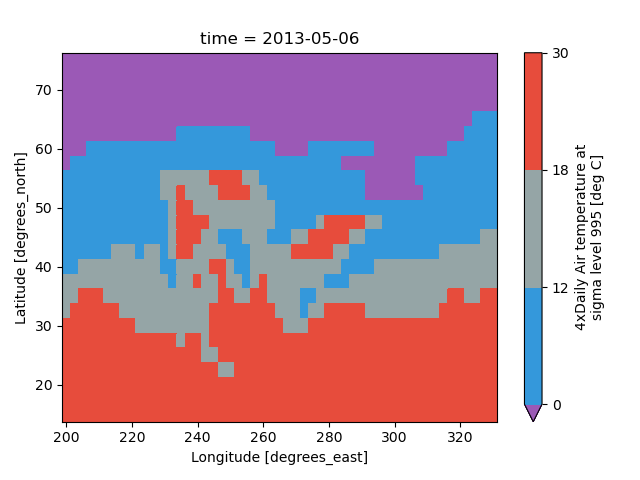Finally, if you have Seaborn installed, you can also specify a seaborn color palette to the cmap argument. Note that levels must be specified with seaborn color palettes if using imshow or pcolormesh (but not with contour or contourf, since levels are chosen automatically).

In : air2d.plot(levels=10, cmap="husl")

In : plt.draw()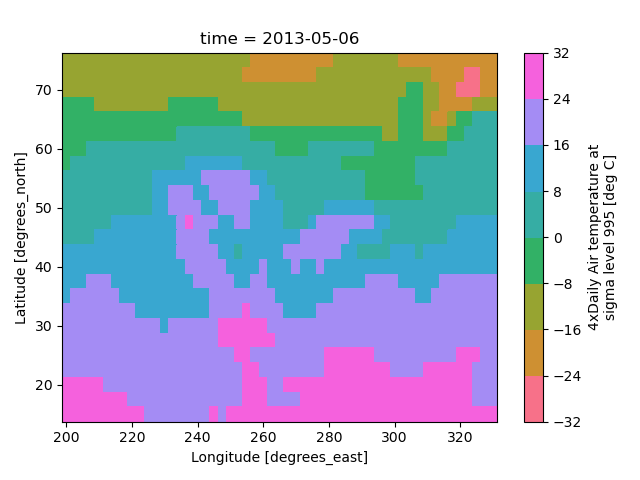### Faceting¶

Faceting here refers to splitting an array along one or two dimensions and plotting each group. xarray’s basic plotting is useful for plotting two dimensional arrays. What about three or four dimensional arrays? That’s where facets become helpful. The general approach to plotting here is called “small multiples”, where the same kind of plot is repeated multiple times, and the specific use of small multiples to display the same relationship conditioned on one ore more other variables is often called a “trellis plot”.

Consider the temperature data set. There are 4 observations per day for two years which makes for 2920 values along the time dimension. One way to visualize this data is to make a separate plot for each time period.

The faceted dimension should not have too many values; faceting on the time dimension will produce 2920 plots. That’s too much to be helpful. To handle this situation try performing an operation that reduces the size of the data in some way. For example, we could compute the average air temperature for each month and reduce the size of this dimension from 2920 -> 12. A simpler way is to just take a slice on that dimension. So let’s use a slice to pick 6 times throughout the first year.

In : t = air.isel(time=slice(0, 365 * 4, 250))

In : t.coords
Out:
Coordinates:
* lat      (lat) float32 75.0 72.5 70.0 67.5 65.0 ... 25.0 22.5 20.0 17.5 15.0
* lon      (lon) float32 200.0 202.5 205.0 207.5 ... 322.5 325.0 327.5 330.0
* time     (time) datetime64[ns] 2013-01-01 ... 2013-11-09T12:00:00


#### Simple Example¶

The easiest way to create faceted plots is to pass in row or col arguments to the xarray plotting methods/functions. This returns a xarray.plot.FacetGrid object.

In : g_simple = t.plot(x="lon", y="lat", col="time", col_wrap=3)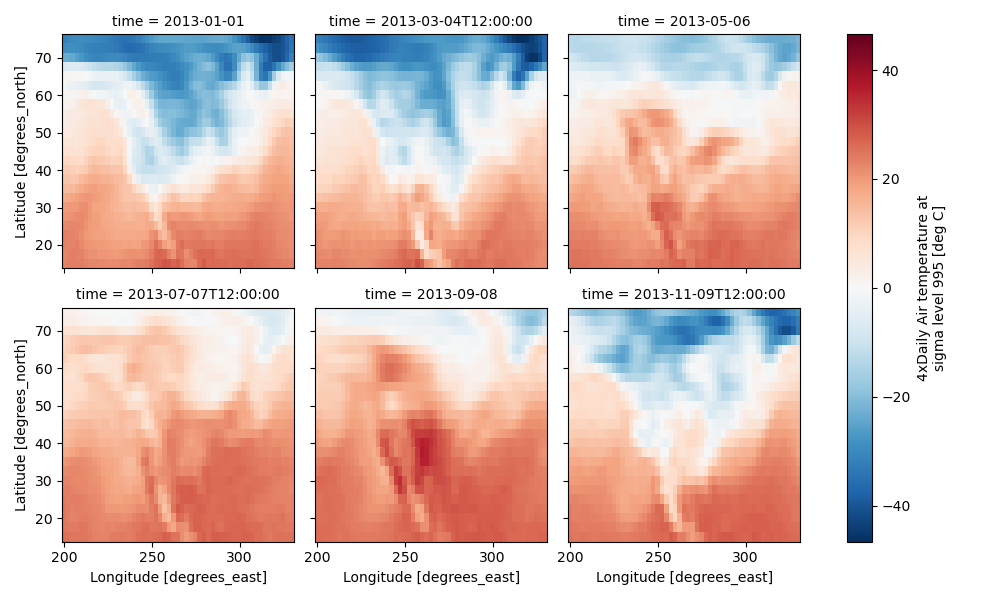Faceting also works for line plots.

In : g_simple_line = t.isel(lat=slice(0, None, 4)).plot(
....:     x="lon", hue="lat", col="time", col_wrap=3
....: )
....: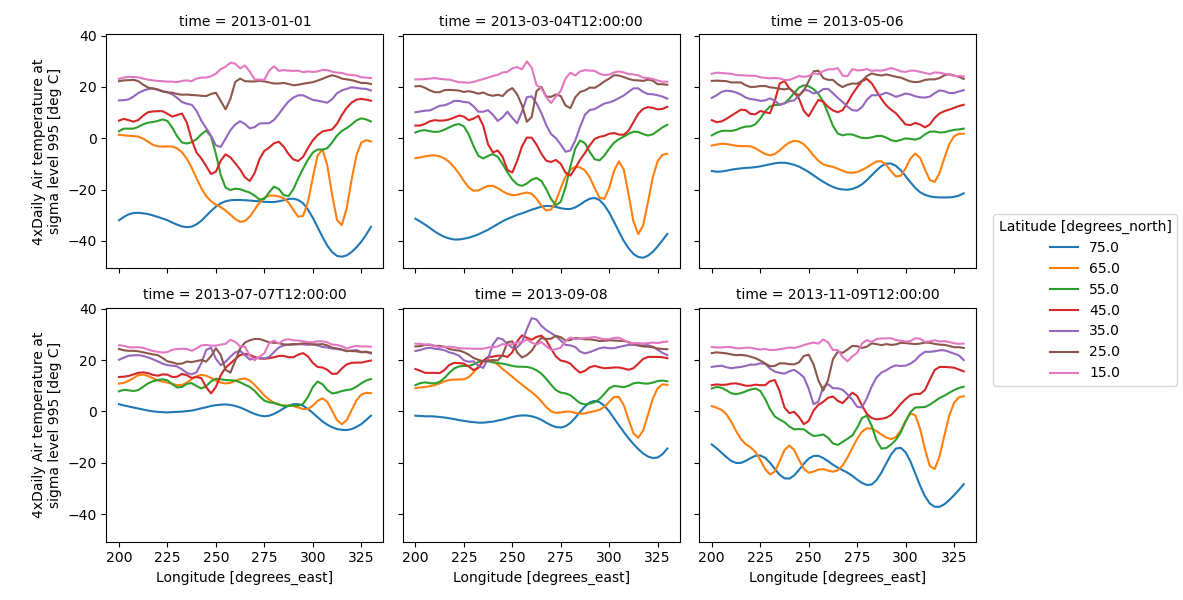#### 4 dimensional¶

For 4 dimensional arrays we can use the rows and columns of the grids. Here we create a 4 dimensional array by taking the original data and adding a fixed amount. Now we can see how the temperature maps would compare if one were much hotter.

In : t2 = t.isel(time=slice(0, 2))

In : t4d = xr.concat([t2, t2 + 40], pd.Index(["normal", "hot"], name="fourth_dim"))

# This is a 4d array
In : t4d.coords
Out:
Coordinates:
* lat         (lat) float32 75.0 72.5 70.0 67.5 65.0 ... 22.5 20.0 17.5 15.0
* lon         (lon) float32 200.0 202.5 205.0 207.5 ... 325.0 327.5 330.0
* time        (time) datetime64[ns] 2013-01-01 2013-03-04T12:00:00
* fourth_dim  (fourth_dim) object 'normal' 'hot'

In : t4d.plot(x="lon", y="lat", col="time", row="fourth_dim")
Out: <xarray.plot.facetgrid.FacetGrid at 0x7f8325f95bb0>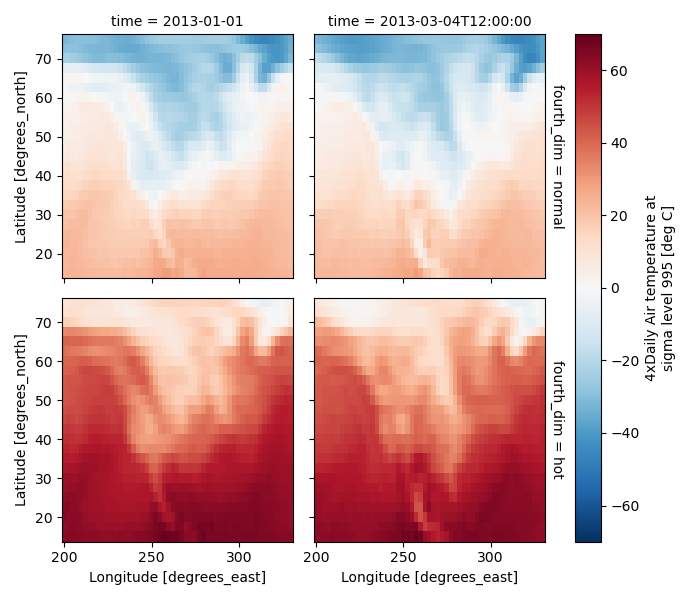#### Other features¶

Faceted plotting supports other arguments common to xarray 2d plots.

In : hasoutliers = t.isel(time=slice(0, 5)).copy()

In : hasoutliers[0, 0, 0] = -100

In : hasoutliers[-1, -1, -1] = 400

In : g = hasoutliers.plot.pcolormesh(
....:     "lon",
....:     "lat",
....:     col="time",
....:     col_wrap=3,
....:     robust=True,
....:     cmap="viridis",
....:     cbar_kwargs={"label": "this has outliers"},
....: )
....: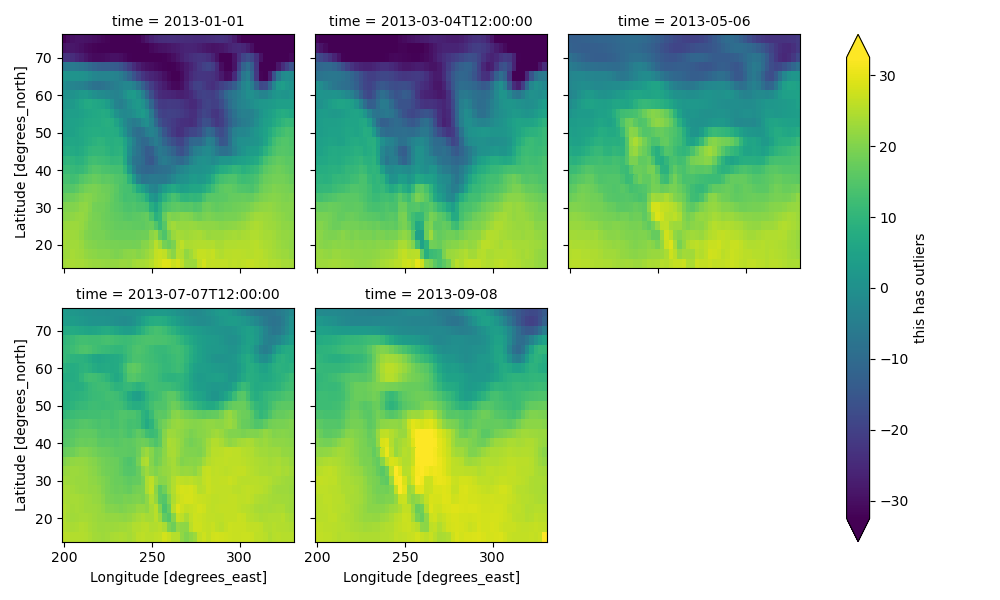#### FacetGrid Objects¶

The object returned, g in the above examples, is a FacetGrid object that links a DataArray to a matplotlib figure with a particular structure. This object can be used to control the behavior of the multiple plots. It borrows an API and code from Seaborn’s FacetGrid. The structure is contained within the axes and name_dicts attributes, both 2d Numpy object arrays.

In : g.axes
Out:
array([[<AxesSubplot:title={'center':'time = 2013-01-01'}, ylabel='Latitude [degrees_north]'>,
<AxesSubplot:title={'center':'time = 2013-03-04T12:00:00'}>,
<AxesSubplot:title={'center':'time = 2013-05-06'}>],
[<AxesSubplot:title={'center':'time = 2013-07-07T12:00:00'}, xlabel='Longitude [degrees_east]', ylabel='Latitude [degrees_north]'>,
<AxesSubplot:title={'center':'time = 2013-09-08'}, xlabel='Longitude [degrees_east]'>,
<AxesSubplot:xlabel='Longitude [degrees_east]'>]], dtype=object)

In : g.name_dicts
Out:
array([[{'time': numpy.datetime64('2013-01-01T00:00:00.000000000')},
{'time': numpy.datetime64('2013-03-04T12:00:00.000000000')},
{'time': numpy.datetime64('2013-05-06T00:00:00.000000000')}],
[{'time': numpy.datetime64('2013-07-07T12:00:00.000000000')},
{'time': numpy.datetime64('2013-09-08T00:00:00.000000000')},
None]], dtype=object)


It’s possible to select the xarray.DataArray or xarray.Dataset corresponding to the FacetGrid through the name_dicts.

In : g.data.loc[g.name_dicts[0, 0]]
Out:
<xarray.DataArray 'air' (lat: 25, lon: 53)>
array([[-100.      ,  -30.649994,  -29.649994, ...,  -40.350006,
-37.649994,  -34.550003],
[ -29.350006,  -28.649994,  -28.449997, ...,  -40.350006,
-37.850006,  -33.850006],
[ -23.149994,  -23.350006,  -24.259995, ...,  -39.949997,
-36.759995,  -31.449997],
...,
[  23.450012,   23.049988,   23.25    , ...,   22.25    ,
21.950012,   21.549988],
[  22.75    ,   23.049988,   23.640015, ...,   22.75    ,
22.75    ,   22.049988],
[  23.140015,   23.640015,   23.950012, ...,   23.75    ,
23.640015,   23.450012]], dtype=float32)
Coordinates:
* lat      (lat) float32 75.0 72.5 70.0 67.5 65.0 ... 25.0 22.5 20.0 17.5 15.0
* lon      (lon) float32 200.0 202.5 205.0 207.5 ... 322.5 325.0 327.5 330.0
time     datetime64[ns] 2013-01-01
Attributes:
long_name:     4xDaily Air temperature at sigma level 995
units:         deg C
precision:     2
GRIB_id:       11
GRIB_name:     TMP
var_desc:      Air temperature
dataset:       NMC Reanalysis
level_desc:    Surface
statistic:     Individual Obs
parent_stat:   Other
actual_range:  [185.16 322.1 ]


Here is an example of using the lower level API and then modifying the axes after they have been plotted.

In : g = t.plot.imshow("lon", "lat", col="time", col_wrap=3, robust=True)

In : for i, ax in enumerate(g.axes.flat):
....:     ax.set_title("Air Temperature %d" % i)
....:

In : bottomright = g.axes[-1, -1]

In : bottomright.annotate("bottom right", (240, 40))
Out: Text(240, 40, 'bottom right')

In : plt.draw()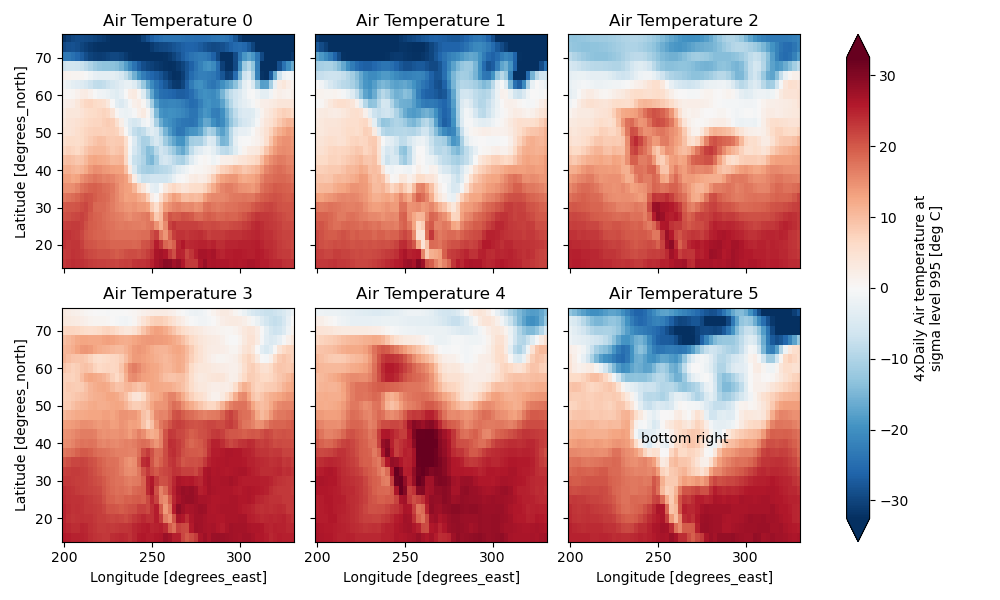FacetGrid objects have methods that let you customize the automatically generated axis labels, axis ticks and plot titles. See set_titles(), set_xlabels(), set_ylabels() and set_ticks() for more information. Plotting functions can be applied to each subset of the data by calling map_dataarray() or to each subplot by calling map().

TODO: add an example of using the map method to plot dataset variables (e.g., with plt.quiver).

## Datasets¶

xarray has limited support for plotting Dataset variables against each other. Consider this dataset

In : ds = xr.tutorial.scatter_example_dataset()

In : ds
Out:
<xarray.Dataset>
Dimensions:  (w: 4, x: 3, y: 11, z: 4)
Coordinates:
* x        (x) int64 0 1 2
* y        (y) float64 0.0 0.1 0.2 0.3 0.4 0.5 0.6 0.7 0.8 0.9 1.0
* z        (z) int64 0 1 2 3
* w        (w) <U5 'one' 'two' 'three' 'five'
Data variables:
A        (x, y, z, w) float64 -0.104 0.02719 -0.0425 ... -0.1175 -0.0183
B        (x, y, z, w) float64 0.0 0.0 0.0 0.0 ... 1.369 1.408 1.387 1.417


Suppose we want to scatter A against B

In : ds.plot.scatter(x="A", y="B")
Out: <matplotlib.collections.PathCollection at 0x7f8325bb3d30>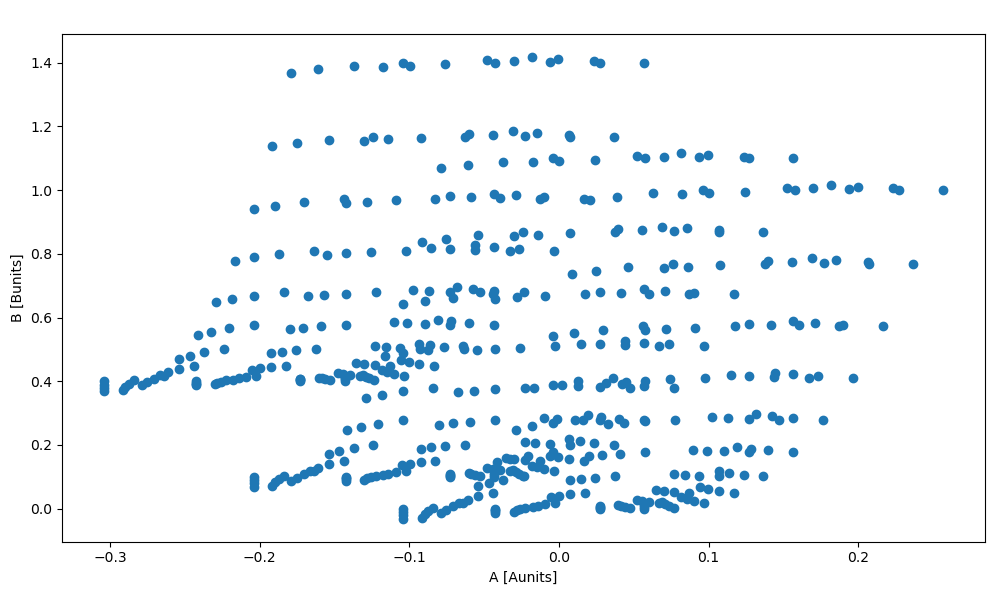The hue kwarg lets you vary the color by variable value

In : ds.plot.scatter(x="A", y="B", hue="w")
Out:
[<matplotlib.collections.PathCollection at 0x7f832616a820>,
<matplotlib.collections.PathCollection at 0x7f8325abb520>,
<matplotlib.collections.PathCollection at 0x7f8325abbd00>,
<matplotlib.collections.PathCollection at 0x7f8325abbeb0>]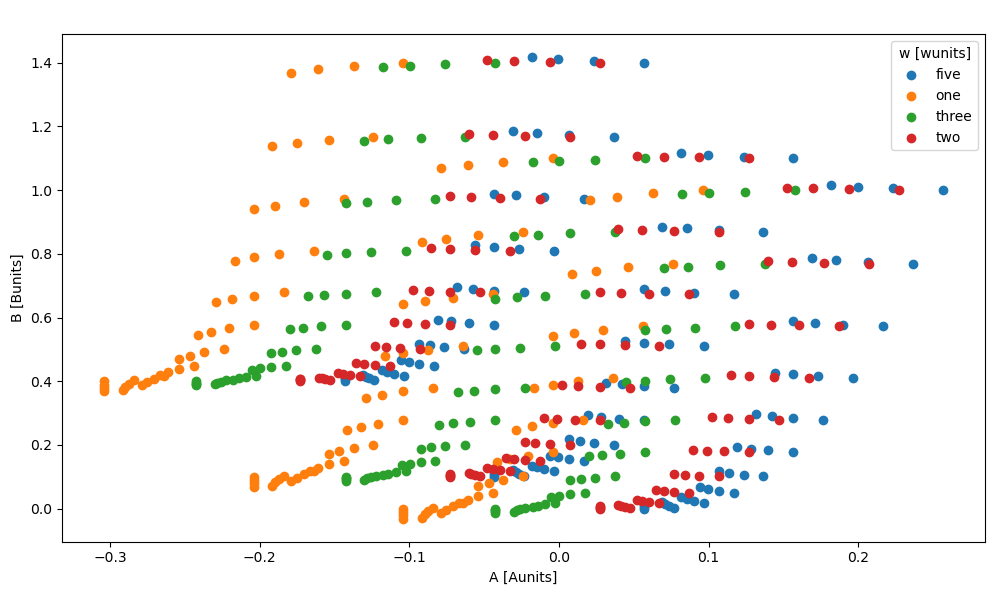When hue is specified, a colorbar is added for numeric hue DataArrays by default and a legend is added for non-numeric hue DataArrays (as above). You can force a legend instead of a colorbar by setting hue_style='discrete'. Additionally, the boolean kwarg add_guide can be used to prevent the display of a legend or colorbar (as appropriate).

In : ds = ds.assign(w=[1, 2, 3, 5])

In : ds.plot.scatter(x="A", y="B", hue="w", hue_style="discrete")
Out:
[<matplotlib.collections.PathCollection at 0x7f8325b72e50>,
<matplotlib.collections.PathCollection at 0x7f8335ca27c0>,
<matplotlib.collections.PathCollection at 0x7f8335c81f10>,
<matplotlib.collections.PathCollection at 0x7f8335ca2c10>]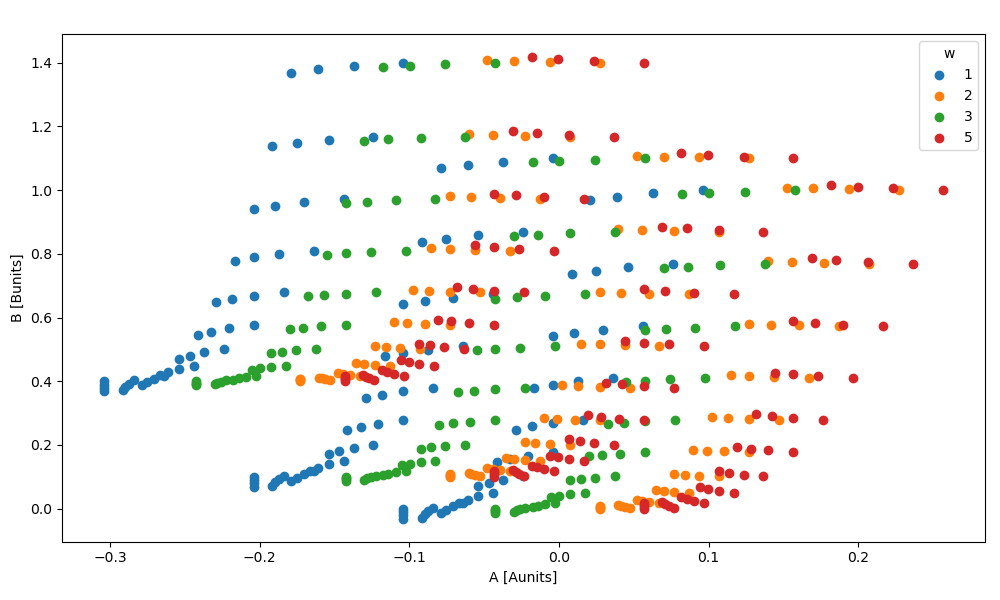The markersize kwarg lets you vary the point’s size by variable value. You can additionally pass size_norm to control how the variable’s values are mapped to point sizes.

In : ds.plot.scatter(x="A", y="B", hue="z", hue_style="discrete", markersize="z")
Out:
[<matplotlib.collections.PathCollection at 0x7f8335c75e20>,
<matplotlib.collections.PathCollection at 0x7f8335c75e50>,
<matplotlib.collections.PathCollection at 0x7f8325b728b0>,
<matplotlib.collections.PathCollection at 0x7f8335c049d0>]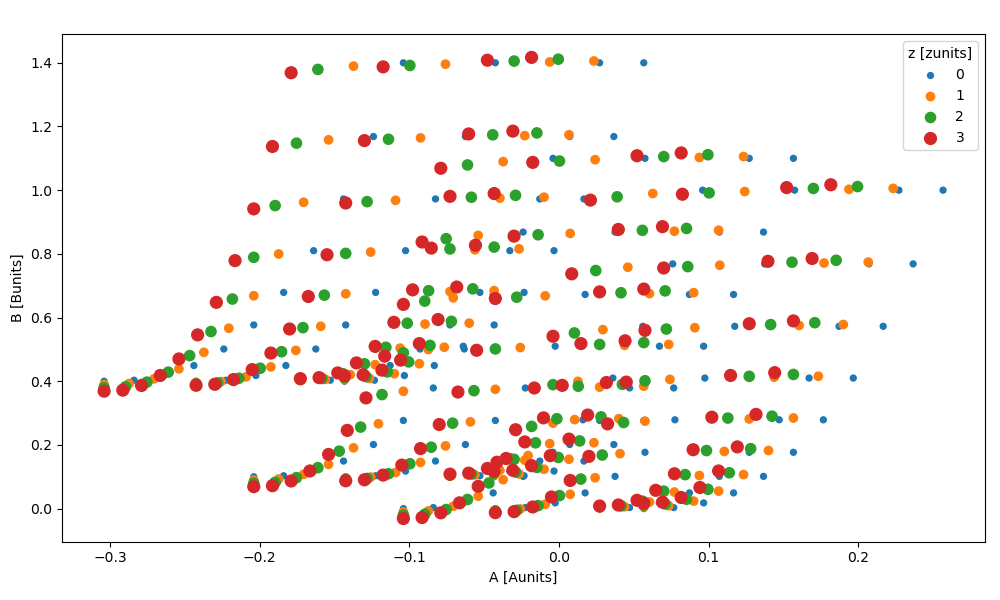Faceting is also possible

In : ds.plot.scatter(x="A", y="B", col="x", row="z", hue="w", hue_style="discrete")
Out: <xarray.plot.facetgrid.FacetGrid at 0x7f8335c6a880>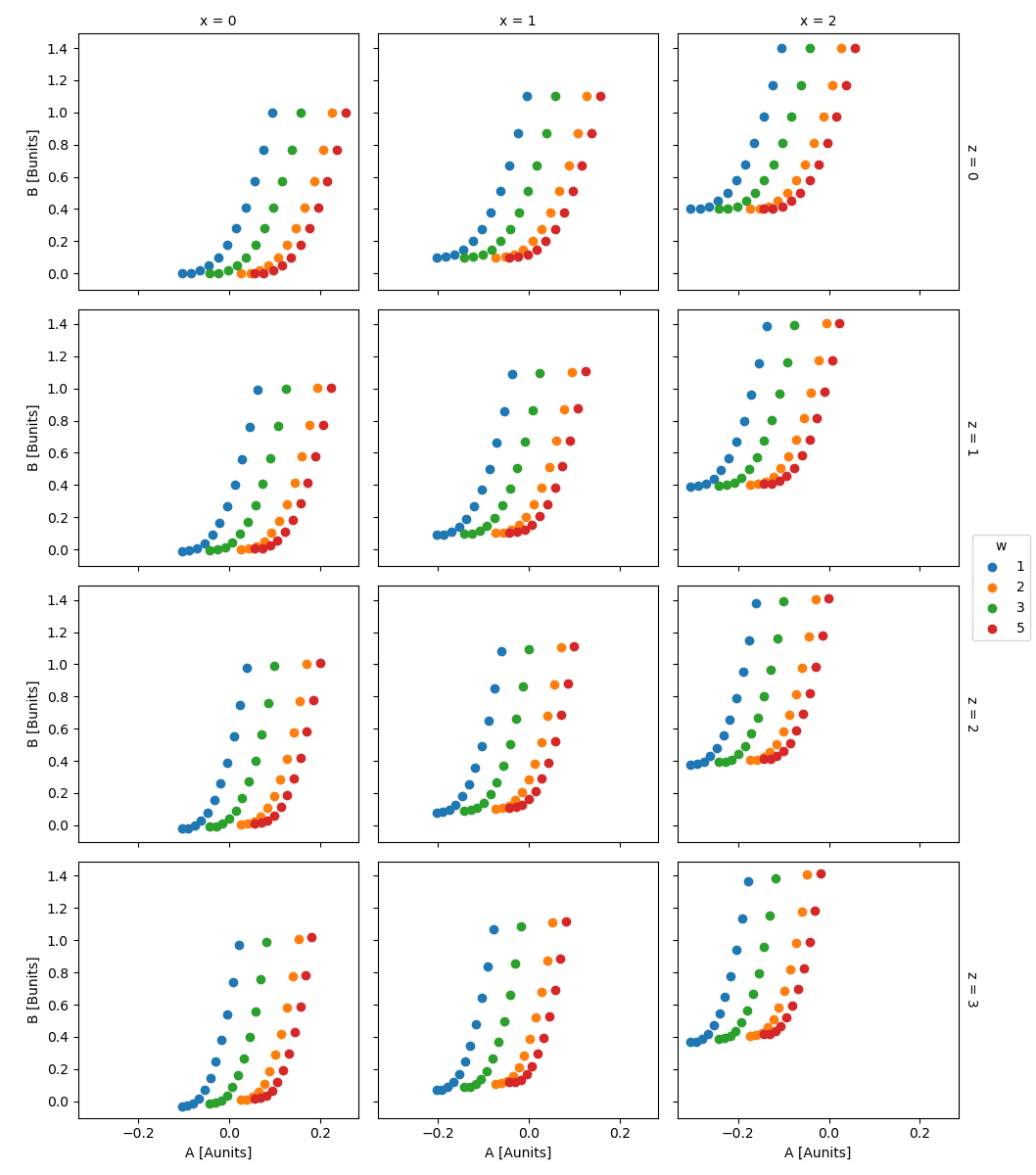For more advanced scatter plots, we recommend converting the relevant data variables to a pandas DataFrame and using the extensive plotting capabilities of seaborn.

## Maps¶

To follow this section you’ll need to have Cartopy installed and working.

This script will plot the air temperature on a map.

In : import cartopy.crs as ccrs

In : air = xr.tutorial.open_dataset("air_temperature").air

In : p = air.isel(time=0).plot(
.....:     subplot_kws=dict(projection=ccrs.Orthographic(-80, 35), facecolor="gray"),
.....:     transform=ccrs.PlateCarree(),
.....: )
.....:

In : p.axes.set_global()

In : p.axes.coastlines()
Out: <cartopy.mpl.feature_artist.FeatureArtist at 0x7f8335a32a60>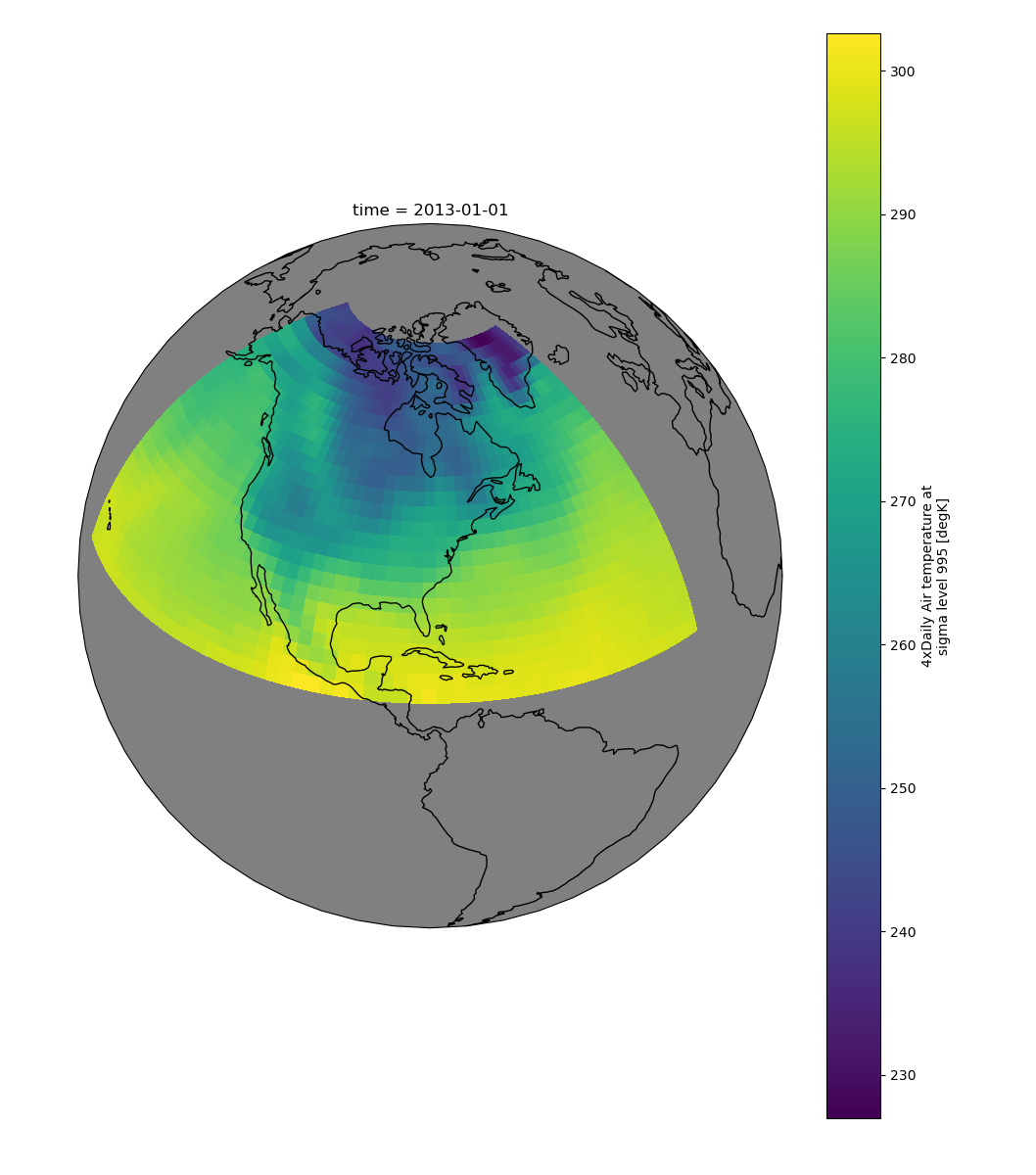When faceting on maps, the projection can be transferred to the plot function using the subplot_kws keyword. The axes for the subplots created by faceting are accessible in the object returned by plot:

In : p = air.isel(time=[0, 4]).plot(
.....:     transform=ccrs.PlateCarree(),
.....:     col="time",
.....:     subplot_kws={"projection": ccrs.Orthographic(-80, 35)},
.....: )
.....:

In : for ax in p.axes.flat:
.....:     ax.coastlines()
.....:     ax.gridlines()
.....:

In : plt.draw()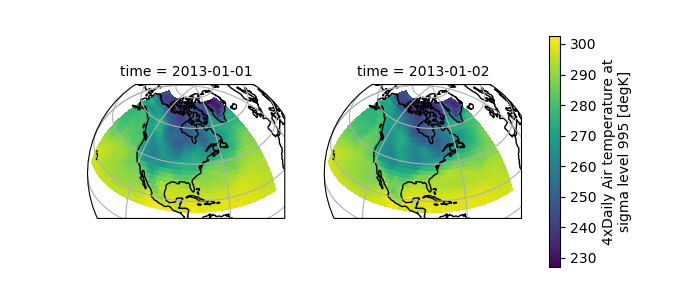## Details¶

### Ways to Use¶

There are three ways to use the xarray plotting functionality:

1. Use plot as a convenience method for a DataArray.

2. Access a specific plotting method from the plot attribute of a DataArray.

3. Directly from the xarray plot submodule.

These are provided for user convenience; they all call the same code.

In : import xarray.plot as xplt

In : da = xr.DataArray(range(5))

In : fig, axes = plt.subplots(ncols=2, nrows=2)

In : da.plot(ax=axes[0, 0])
Out: [<matplotlib.lines.Line2D at 0x7f8335742d60>]

In : da.plot.line(ax=axes[0, 1])
Out: [<matplotlib.lines.Line2D at 0x7f8335912850>]

In : xplt.plot(da, ax=axes[1, 0])
Out: [<matplotlib.lines.Line2D at 0x7f834d4eeeb0>]

In : xplt.line(da, ax=axes[1, 1])
Out: [<matplotlib.lines.Line2D at 0x7f83357a2190>]

In : plt.tight_layout()

In : plt.draw()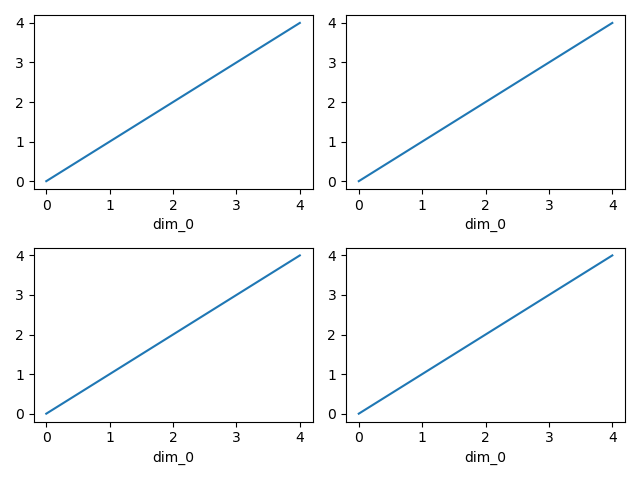Here the output is the same. Since the data is 1 dimensional the line plot was used.

The convenience method xarray.DataArray.plot() dispatches to an appropriate plotting function based on the dimensions of the DataArray and whether the coordinates are sorted and uniformly spaced. This table describes what gets plotted:

 Dimensions Plotting function 1 xarray.plot.line() 2 xarray.plot.pcolormesh() Anything else xarray.plot.hist()

### Coordinates¶

If you’d like to find out what’s really going on in the coordinate system, read on.

In : a0 = xr.DataArray(np.zeros((4, 3, 2)), dims=("y", "x", "z"), name="temperature")

In : a0[0, 0, 0] = 1

In : a = a0.isel(z=0)

In : a
Out:
<xarray.DataArray 'temperature' (y: 4, x: 3)>
array([[1., 0., 0.],
[0., 0., 0.],
[0., 0., 0.],
[0., 0., 0.]])
Dimensions without coordinates: y, x


The plot will produce an image corresponding to the values of the array. Hence the top left pixel will be a different color than the others. Before reading on, you may want to look at the coordinates and think carefully about what the limits, labels, and orientation for each of the axes should be.

In : a.plot()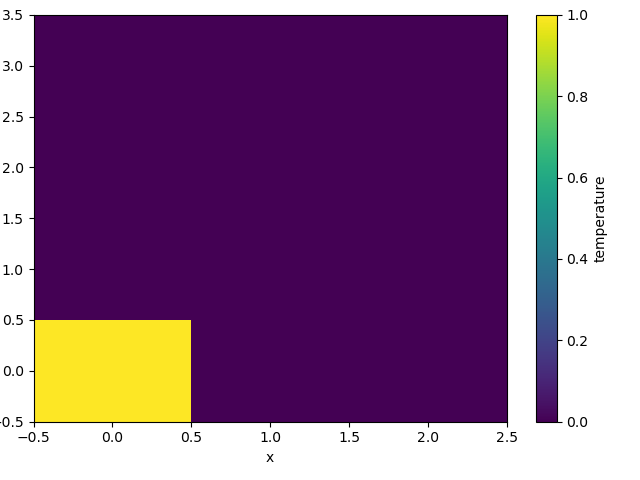It may seem strange that the values on the y axis are decreasing with -0.5 on the top. This is because the pixels are centered over their coordinates, and the axis labels and ranges correspond to the values of the coordinates.

### Multidimensional coordinates¶

You can plot irregular grids defined by multidimensional coordinates with xarray, but you’ll have to tell the plot function to use these coordinates instead of the default ones:

In : lon, lat = np.meshgrid(np.linspace(-20, 20, 5), np.linspace(0, 30, 4))

In : lon += lat / 10

In : lat += lon / 10

In : da = xr.DataArray(
.....:     np.arange(20).reshape(4, 5),
.....:     dims=["y", "x"],
.....:     coords={"lat": (("y", "x"), lat), "lon": (("y", "x"), lon)},
.....: )
.....:

In : da.plot.pcolormesh("lon", "lat")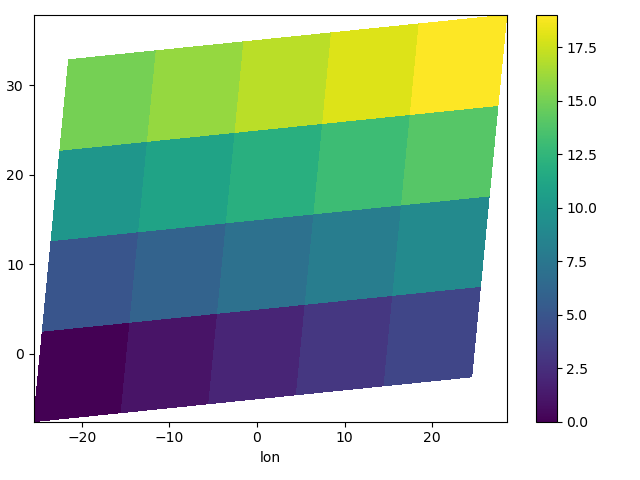Note that in this case, xarray still follows the pixel centered convention. This might be undesirable in some cases, for example when your data is defined on a polar projection (GH781). This is why the default is to not follow this convention when plotting on a map:

In : import cartopy.crs as ccrs

In : ax = plt.subplot(projection=ccrs.PlateCarree())

In : da.plot.pcolormesh("lon", "lat", ax=ax)

In : ax.scatter(lon, lat, transform=ccrs.PlateCarree())
Out: <matplotlib.collections.PathCollection at 0x7f833565d280>

In : ax.coastlines()
Out: <cartopy.mpl.feature_artist.FeatureArtist at 0x7f833568f340>

In : ax.gridlines(draw_labels=True)
Out: <cartopy.mpl.gridliner.Gridliner at 0x7f8335665400>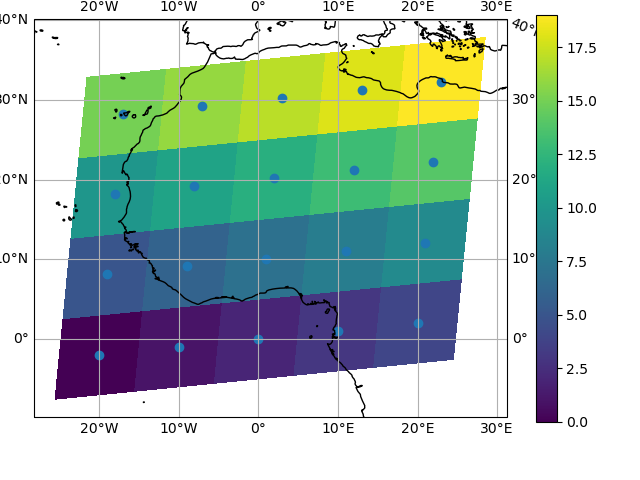You can however decide to infer the cell boundaries and use the infer_intervals keyword:

In : ax = plt.subplot(projection=ccrs.PlateCarree())

In : da.plot.pcolormesh("lon", "lat", ax=ax, infer_intervals=True)

In : ax.scatter(lon, lat, transform=ccrs.PlateCarree())
Out: <matplotlib.collections.PathCollection at 0x7f8335531610>

In : ax.coastlines()
Out: <cartopy.mpl.feature_artist.FeatureArtist at 0x7f8335531370>

In : ax.gridlines(draw_labels=True)
Out: <cartopy.mpl.gridliner.Gridliner at 0x7f833563a0a0>Note

The data model of xarray does not support datasets with cell boundaries yet. If you want to use these coordinates, you’ll have to make the plots outside the xarray framework.

One can also make line plots with multidimensional coordinates. In this case, hue must be a dimension name, not a coordinate name.

In : f, ax = plt.subplots(2, 1)

In : da.plot.line(x="lon", hue="y", ax=ax)
Out:
[<matplotlib.lines.Line2D at 0x7f83354cc1c0>,
<matplotlib.lines.Line2D at 0x7f83354cc2b0>,
<matplotlib.lines.Line2D at 0x7f83354cc370>,
<matplotlib.lines.Line2D at 0x7f83354cc430>]

In : da.plot.line(x="lon", hue="x", ax=ax)
Out:
[<matplotlib.lines.Line2D at 0x7f833541c340>,
<matplotlib.lines.Line2D at 0x7f83354fef40>,
<matplotlib.lines.Line2D at 0x7f83354fe0a0>,
<matplotlib.lines.Line2D at 0x7f83354bc0a0>,
<matplotlib.lines.Line2D at 0x7f83354bc160>]